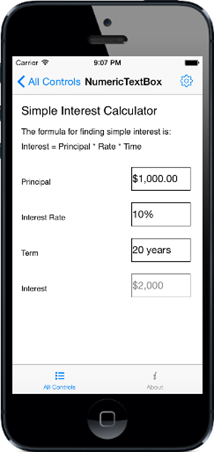# Getting Started with Xamarin.iOS SfNumericTextBox

17 Jul 20201 minute to read

This section explains you the steps to configure a numeric textbox control in a real-time scenario and also provides a walk-through on some of the customization features available in numeric textbox control.

## Reference Essential Studio Components in your Solution

After installing Essential Studio for Xamarin, you can find all the required assemblies in the installation folders, typically:

{Syncfusion Installed location}\Essential Studio{version number}\lib

NOTE

Assemblies are available in unzipped package location in Mac

and below assembly reference to the iOS unified project.

iOS-unifed\Syncfusion.SfNumericTextBox.iOS.dll

• C#
• ``````[C#]

using Syncfusion.SfNumericTextBox.iOS;``````
• Now add the SfNumericTextBox control with a required optimal name by using the included namespace.
• C#
• ``````[C#]

SfNumericTextBox numericTextBox = new SfNumericTextBox()
{
Frame = new CGRect(10, 50, 350, 40),
};

## Enable Parsing Mode

SfNumericTextBox provides option to display the value in double or decimal. Following code shows the Decimal parsing mode which can be set through `ParserMode` property.

• C#
• ``````[C#]

numericTextBox.ParserMode = SFNumericTextBoxParsers.Decimal;``````

## Configuring properties

Format string, value and maximum number of decimal digits can be customized in SfNumericTextBox as below.

• C#
• ``````[C#]

SfNumericTextBox numericTextBox = new SfNumericTextBox()
{
Value = 1000,
Watermark = "Principal Amount",
MaximumNumberDecimalDigits = 2,
FormatString = "c",
AllowNull = true,
CultureInfo = new NSLocale("en_us"),
PercentDisplayMode = SFNumericTextBoxPercentDisplayMode.Compute
};``````You can find the complete getting started sample from this link.